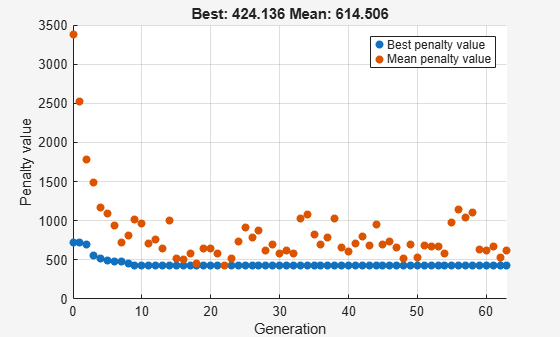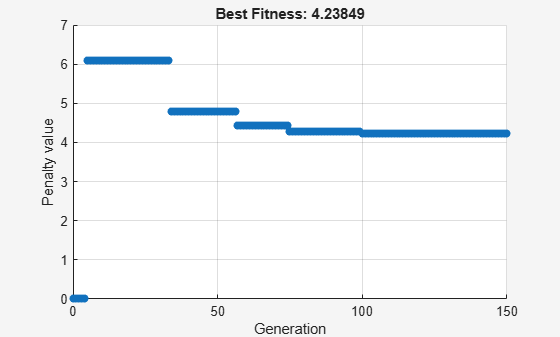## Mixed Integer `ga` Optimization

### Solving Mixed Integer Optimization Problems

`ga` can solve problems when certain variables are integer-valued. Give `intcon`, a vector of the x components that are integers:

```[x,fval,exitflag] = ga(fitnessfcn,nvars,A,b,[],[],... lb,ub,nonlcon,intcon,options)```

`intcon` is a vector of positive integers that contains the x components that are integer-valued. For example, if you want to restrict `x(2)` and `x(10)` to be integers, set `intcon` to `[2,10]`.

The `surrogateopt` solver also accepts integer constraints.

Note

Restrictions exist on the types of problems that `ga` can solve with integer variables. In particular, `ga` does not accept nonlinear equality constraints when there are integer variables. For details, see Characteristics of the Integer ga Solver.

Tip

`ga` solves integer problems best when you provide lower and upper bounds for every x component.

#### Mixed Integer Optimization of Rastrigin's Function

This example shows how to find the minimum of Rastrigin's function restricted so the first component of x is an integer. The components of x are further restricted to be in the region $5\pi \le x\left(1\right)\le 20\pi ,\phantom{\rule{0.5em}{0ex}}-20\pi \le x\left(2\right)\le -4\pi$ .

Set up the bounds for your problem

```lb = [5*pi,-20*pi]; ub = [20*pi,-4*pi];```

Set a plot function so you can view the progress of ga

`opts = optimoptions('ga','PlotFcn',@gaplotbestf);`

Call the ga solver where x(1) has integer values

```rng(1,'twister') % for reproducibility intcon = 1; [x,fval,exitflag] = ga(@rastriginsfcn,2,[],[],[],[],... lb,ub,[],intcon,opts)``````Optimization terminated: average change in the penalty fitness value less than options.FunctionTolerance and constraint violation is less than options.ConstraintTolerance. ```
```x = 1×2 16.0000 -12.9325 ```
```fval = 424.1355 ```
```exitflag = 1 ```

ga converges quickly to the solution.

### Characteristics of the Integer ga Solver

There are some restrictions on the types of problems that `ga` can solve when you include integer constraints:

• No nonlinear equality constraints. Any nonlinear constraint function must return `[]` for the nonlinear equality constraint. For a possible workaround, see Integer Programming with a Nonlinear Equality Constraint.

• Only `doubleVector` population type.

• No hybrid function. `ga` overrides any setting of the `HybridFcn` option.

• `ga` ignores the `ParetoFraction`, `DistanceMeasureFcn`, `InitialPenalty`, and `PenaltyFactor` options.

The listed restrictions are mainly natural, not arbitrary. For example, no hybrid functions support integer constraints. So `ga` does not use hybrid functions when there are integer constraints.

#### Integer Programming with a Nonlinear Equality Constraint

This example attempts to locate the minimum of the Ackley function (included when you run this example) in five dimensions with these constraints:

• `x(1)`, `x(3)`, and `x(5)` are integers.

• `norm(x) = 4`.

The `ga` solver does not support nonlinear equality constraints, only nonlinear inequality constraints. This example shows a workaround that applies for some problems, but is not guaranteed to work.

The Ackley function is difficult to minimize. Adding integer and equality constraints increases the difficulty.

To include the nonlinear equality constraint, give a small tolerance `tol` that allows the norm of x to be within `tol` of 4. Without a tolerance, the nonlinear equality constraint is never satisfied, and the solver does not reach a feasible solution.

Write the expression `norm(x) = 4` as two “less than zero” inequalities.

`$‖x-4‖\le 0$`

$-‖x-4‖\le 0$.

Allow a small tolerance in the inequalities.

`$‖x-4‖-tol\le 0$`

$-‖x-4‖-tol\le 0$.

Write a nonlinear inequality constraint function `eqCon` that implements these inequalities.

`type eqCon`
```function [c, ceq] = eqCon(x) ceq = []; rad = 4; tol = 1e-3; confcnval = norm(x) - rad; c = [confcnval - tol;-confcnval - tol]; ```

Set these options:

• `MaxStallGenerations` = 50 — Allow the solver to try for a while.

• `FunctionTolerance` = 1e-10 — Specify a stricter stopping criterion than usual.

• `MaxGenerations` = 500 — Allow more generations than default.

• `PlotFcn` = `@gaplotbestfun` — Observe the optimization.

```opts = optimoptions('ga','MaxStallGenerations',50,'FunctionTolerance',1e-10,... 'MaxGenerations',500,'PlotFcn',@gaplotbestfun);```

Set lower and upper bounds to help the solver.

```nVar = 5; lb = -5*ones(1,nVar); ub = 5*ones(1,nVar);```

Solve the problem.

```rng(0,'twister') % for reproducibility [x,fval,exitflag] = ga(@ackleyfcn,nVar,[],[],[],[], ... lb,ub,@eqCon,[1 3 5],opts);``````Optimization terminated: average change in the penalty fitness value less than options.FunctionTolerance and constraint violation is less than options.ConstraintTolerance. ```

Examine the solution.

`x,fval,exitflag,norm(x)`
```x = 1×5 0 0.9706 1.0000 3.6158 -1.0000 ```
```fval = 5.9676 ```
```exitflag = 1 ```
```ans = 4.0020 ```

The odd `x` components are integers, as specified. The norm of `x` is 4, to within the given relative tolerance of 1e-3.

Despite the positive exit flag, the solution is not the global optimum. Run the problem again with a larger population and examine the solution.

```opts = optimoptions(opts,'Display','off','PopulationSize',400); [x2,fval2,exitflag2] = ga(@ackleyfcn,nVar,[],[],[],[], ... lb,ub,@eqCon,[1 3 5],opts);```Examine the second solution.

`x2,fval2,exitflag2,norm(x2)`
```x2 = 1×5 -1.0000 2.0082 -1.0000 -2.9954 1.0000 ```
```fval2 = 4.2385 ```
```exitflag2 = 1 ```
```ans = 4.0006 ```

The second run gives a better solution (lower fitness function value). Again, the odd `x` components are integers, and the norm of `x2` is 4, to within the given relative tolerance of 1e-3.

Be aware that this procedure can fail; `ga` has difficulty with simultaneous integer and nonlinear equality constraints.

### Effective Integer `ga`

To use `ga` most effectively on integer problems, follow these guidelines.

• Bound each component as tightly as you can. This practice gives `ga` the smallest search space, enabling `ga` to search most effectively.

• If you cannot bound a component, then specify an appropriate initial range. By default, `ga` creates an initial population with range `[-1e4,1e4]` for each component. A smaller or larger initial range can give better results when the default value is inappropriate. To change the initial range, use the `InitialPopulationRange` option.

• If you have more than 10 variables, set a population size that is larger than default by using the `PopulationSize` option. The default value is 200 for six or more variables. For a large population size:

• `ga` can take a long time to converge. If you reach the maximum number of generations (exit flag `0`), increase the value of the `MaxGenerations` option.

• Decrease the mutation rate. To do so, increase the value of the `CrossoverFraction` option from its default of `0.8` to `0.9` or higher.

• Increase the value of the `EliteCount` option from its default of `0.05*PopulationSize` to `0.1*PopulationSize` or higher.

For information on options, see the `ga` `options` input argument.

### Integer `ga` Algorithm

Integer programming with `ga` involves several modifications of the basic algorithm (see How the Genetic Algorithm Works). For integer programming:

• By default, special creation, crossover, and mutation functions enforce variables to be integers. For details, see Deep et al. .

• If you use nondefault creation, crossover, or mutation functions, `ga` enforces linear feasibility and feasibility with respect to integer constraints at each iteration.

• The genetic algorithm attempts to minimize a penalty function, not the fitness function. The penalty function includes a term for infeasibility. This penalty function is combined with binary tournament selection by default to select individuals for subsequent generations. The penalty function value of a member of a population is:

• If the member is feasible, the penalty function is the fitness function.

• If the member is infeasible, the penalty function is the maximum fitness function among feasible members of the population, plus a sum of the constraint violations of the (infeasible) point.

For details of the penalty function, see Deb .

 Deb, Kalyanmoy. An efficient constraint handling method for genetic algorithms. Computer Methods in Applied Mechanics and Engineering, 186(2–4), pp. 311–338, 2000.

 Deep, Kusum, Krishna Pratap Singh, M.L. Kansal, and C. Mohan. A real coded genetic algorithm for solving integer and mixed integer optimization problems. Applied Mathematics and Computation, 212(2), pp. 505–518, 2009.